Next: How FFTEASY works Up: Storage: What Goes Where Previous: Output of Fourier Transforms

### Output of Fourier Transforms of Real Data

For real arrays there is a subtlety in the arrangement of the output. Of course a real function is just a special case of a complex function and you could always take the Fourier transform of an array of real numbers by putting it in an array twice as big with every other component set toand then submitting it to fftc1 or fftcn. This method is obviously quite wasteful. The point of a Fourier transform routine for real data is to take advantage of the fact that N independent input points only produce N independent output points, so even though the result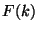consists of complex numbers they can all be stored in the original array. The reason this can be done is because the Fourier transform of a real set of data obeys the symmetry, so it's redundant to store the negative frequency output. You still need the valuesand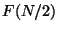but these are both real so they only take up half as much storage as the other frequencies. The net result is thatreal inputs producereal outputs in the form ofcomplex numbers and two real ones.

For fftr1 this makes the output scheme very simple. The first two numbers in the output array are the real valuesand. The rest of the values contain alternate real and imaginary parts forthrough.

For fftrn the story becomes more complicated. If I letstand for the momentum in the last dimension of the array then I don't need to output any negative values of; they can all be recovered by symmetry. On the other hand the values at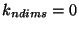and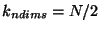are in general no longer real. It is still true that the number of independent outputs equals the number of inputs, but there is no obvious scheme for packing all of the outputs into the original array. Rather than coming up with a complicated scheme that would make it difficult to find different frequency components I simply let the entire plane of valuesbe represented in the original array and store all the values within a separate array. Thus if you look for the values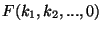andyou will find them both in the output and they will be complex conjugates of each other.

So after all is said and done the output of fftrn is arranged with the last dimension going up fromtoand all the others wrapping around as described for the complex routines above. The values withare stored in the array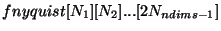.

If you have generated an array using fftr1 or fftrn then you can simply give that array back to the same function to do an inverse transform and recover your original data. If, however, you want to generate data in frequency space and then transform it to position space you have to be careful to ensure that your input obeys the symmetry. For fftr1 this simply means generating real numbers for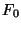andand putting them in the first two slots of the array and then filling all the subsequent slots with complex numbers. For fftrn, however, you have to make sure that for all the values with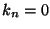(the first plane of values in your lattice) this symmetry is obeyed. You then have to fill fnyquist with the values with, obeying the same symmetry conditions.Next: How FFTEASY works Up: Storage: What Goes Where Previous: Output of Fourier Transforms